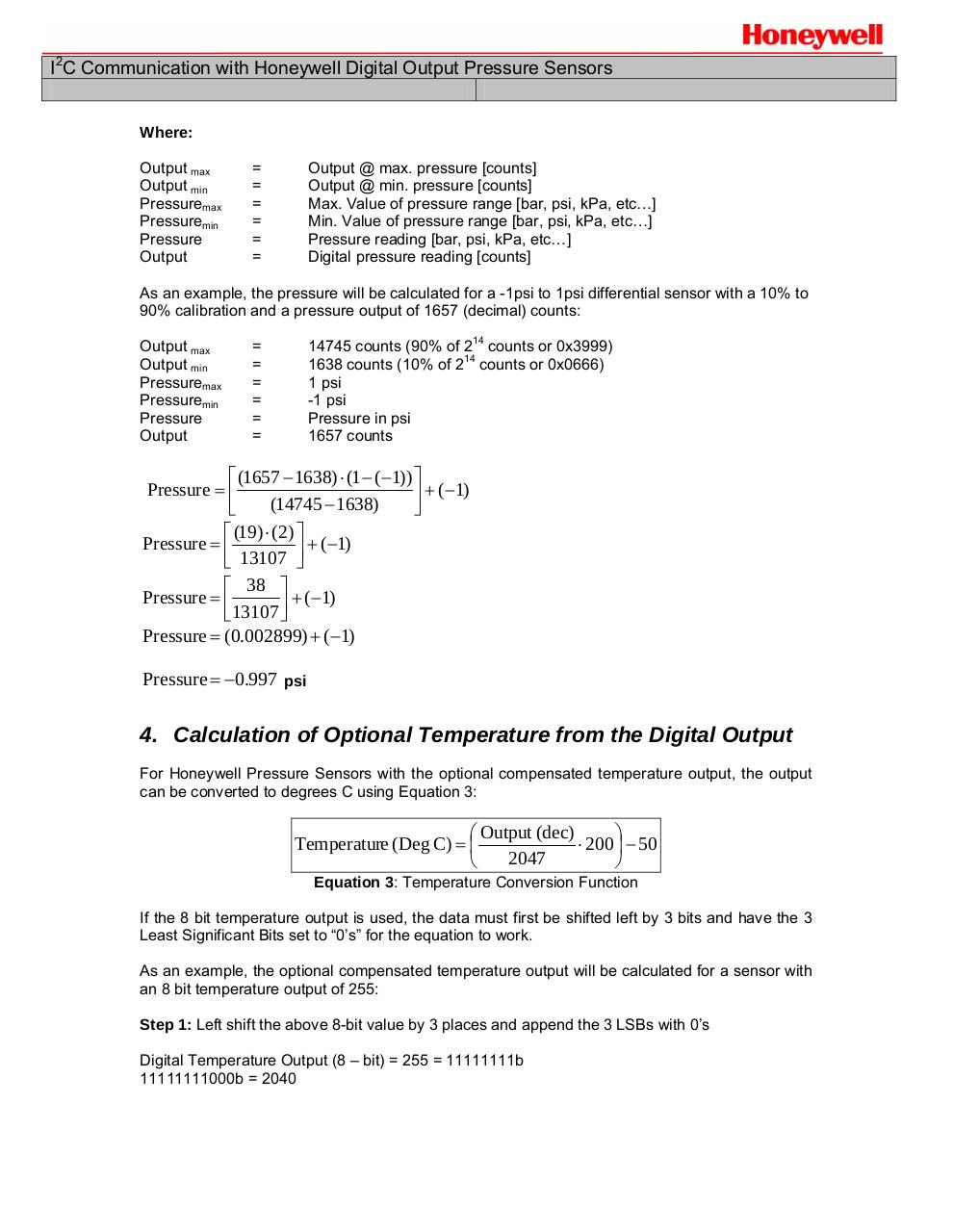# Honeywell.pdfPage 1 2 3 4 5 6

#### Text preview

I2C Communication with Honeywell Digital Output Pressure Sensors

Where:
Output max
Output min
Pressuremax
Pressuremin
Pressure
Output

=
=
=
=
=
=

Output @ max. pressure [counts]
Output @ min. pressure [counts]
Max. Value of pressure range [bar, psi, kPa, etc…]
Min. Value of pressure range [bar, psi, kPa, etc…]
Pressure reading [bar, psi, kPa, etc…]

As an example, the pressure will be calculated for a -1psi to 1psi differential sensor with a 10% to
90% calibration and a pressure output of 1657 (decimal) counts:
Output max
Output min
Pressuremax
Pressuremin
Pressure
Output

14745 counts (90% of 214 counts or 0x3999)
1638 counts (10% of 214 counts or 0x0666)
1 psi
-1 psi
Pressure in psi
1657 counts

=
=
=
=
=
=

⎡ (1657 − 1638) ⋅ (1 − (−1)) ⎤
Pressure = ⎢
⎥ + (−1)
(14745 − 1638)

⎡ (19) ⋅ (2) ⎤
Pressure = ⎢
+ (−1)
⎣ 13107 ⎥⎦
⎡ 38 ⎤
Pressure = ⎢
+ (−1)
⎣13107 ⎥⎦
Pressure = (0.002899) + (−1)

Pressure = −0.997 psi

4. Calculation of Optional Temperature from the Digital Output
For Honeywell Pressure Sensors with the optional compensated temperature output, the output
can be converted to degrees C using Equation 3:

⎛ Output (dec)

Temperature (Deg C) = ⎜
⋅ 200 ⎟ − 50
2047

Equation 3: Temperature Conversion Function
If the 8 bit temperature output is used, the data must first be shifted left by 3 bits and have the 3
Least Significant Bits set to “0’s” for the equation to work.
As an example, the optional compensated temperature output will be calculated for a sensor with
an 8 bit temperature output of 255:
Step 1: Left shift the above 8-bit value by 3 places and append the 3 LSBs with 0’s
Digital Temperature Output (8 – bit) = 255 = 11111111b
11111111000b = 2040